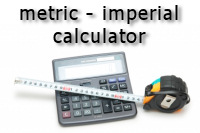# Metric Conversion Calculator - Area, Length, Weight and VolumeWelcome to our Metric Conversion Calculator.

Our calculators are very easy to use. Scroll down to either the imperial to metric or the metric to imperial calculator, enter the value in either the Area, Length, Weight or Volume boxes, select the type of units and click convert.
Note: When entering decimal values use the full stop key . as the decimal point.

## Imperial to metric calculations

Area calculator - square inches / square feet / square yards / acres TO square cm / square metres
to

Length calculator - inches / feet / yards / miles TO mm / cm / metres / kilometres
to

Weight calculator - ounces / pounds / stone / hundredweight / long ton TO grams / kilograms / metric ton
to

Volume calculator - cubic inch / cubic foot / fl ounce / pint / gallon TO cubic cm / cubic metres / ml / litres
to

## Metric to imperial calculations

Area calculator - square cm / square metres TO square inches / square feet / square yards / acres
to

Length calculator - mm / cm / metres / kilometres TO inches / feet / yards / miles
to

Weight calculator - grams / kilograms / metric ton TO ounces / pounds / stone / hundredweight / long ton
to

Volume calculator - cubic cm / cubic metres / ml / litres TO cubic inch / cubic foot / fl ounce / pint / gallon
to